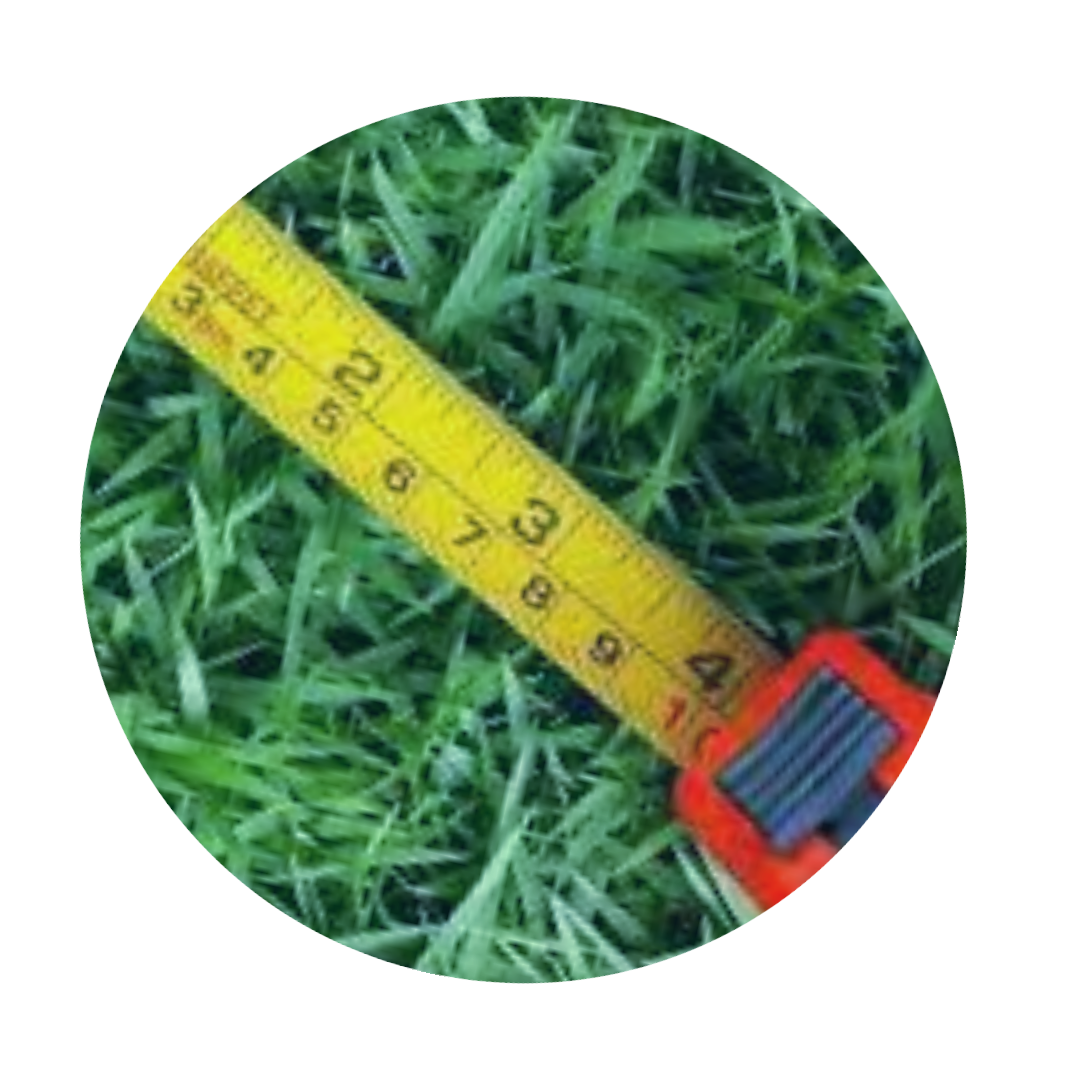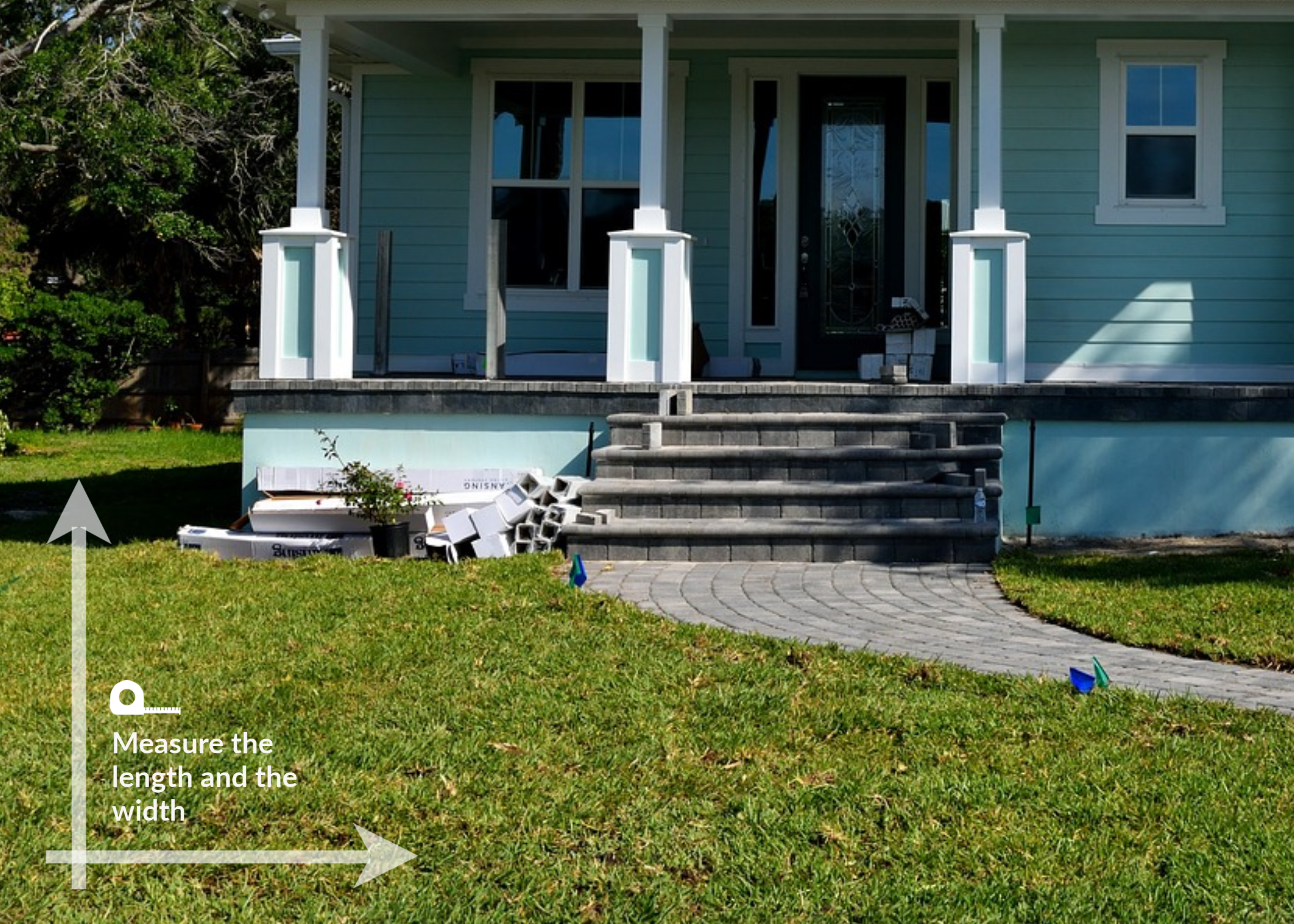# How to Measure for Sod## Looking to sod your yard but unsure how to measure for sod? Let's calculate square feet and how many rolls of grass you need.

First you can use our area calculator here to measure your yard and get an estimate of how much sod you will need.

Secondly you should measure your yard using a measuring tape. To calculate your area in square feet, measure the length and the width of the area you wish to sod and use the sod calculator below.If the area you're wanting to measure has an odd shape, a good way to calculate the overall square footage is by dividing it into smaller individual squares or rectangles, then calculate.

Once you've measured for sod and know how much sod you need your next step is to pick the best grass type.

What type of sod should I get? See our Sod Comparison